Algebra 2 7-5 Guided Practice: Exponential and Logarithmic Equations
starstarstarstarstarstarstarstarstarstar
by Matthew Richardson
| 13 Questions1
10
Solve It! You're a winner on a TV game show. Which prize is the better deal? Explain.

Prize A is the better deal.
Prize A is the better deal for the first 3 weeks, but then Prize B is the better deal.
Prize B is the better deal.
Prize B is the better deal for the first 3 weeks, but then Prize A is the better deal.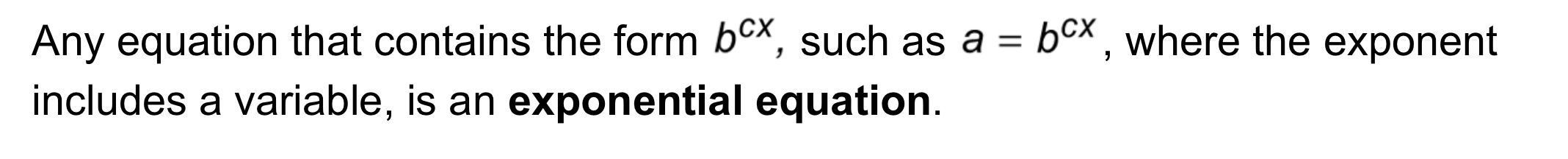2
3
2
10
Take Note: Define exponential equation.
3
10
Take Note: Provide an example of an exponential equation.4
4
10
Problem 1 Got It?
A
B
C
D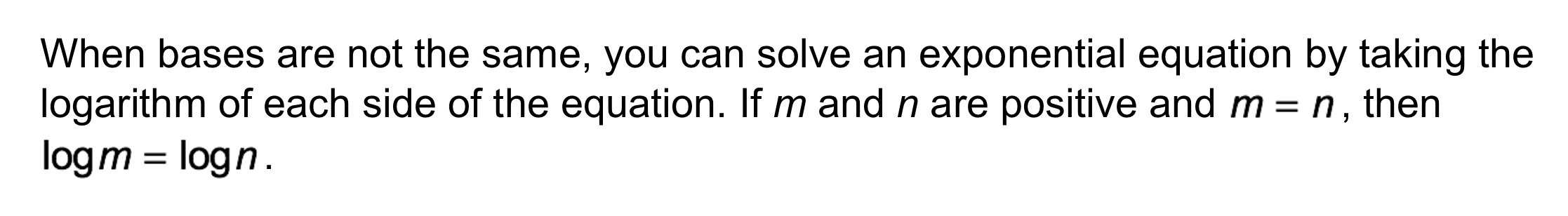5
5
10
Take Note: Describe the process of solving exponential equations with different bases by taking the logarithm of both sides.
6
10
Problem 2 Got It? What is the solution? Round your answer to the hundredths place.

Problem 1 is provided here to help you respond to the Problem 2 Got It? item that follows.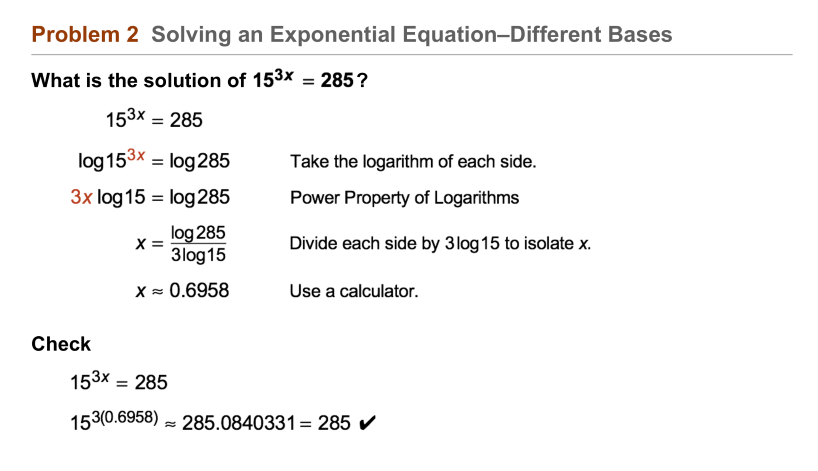7
7
10
Problem 2 Got It? Reasoning: Why can't you use the same method you used in Problem 1 to solve Problem 2?
You can't use the same method because the terms cannot be written with a common base.
You can't use the same method because 3 is a factor of 285.
You can't use the same method because the coefficient of x is odd.8
8
10
Problem 3 Got It?
A
B
C
D9
9
10
Problem 3 Got It?
A
B
C
D10
10
10
Problem 4 Got It? Resource Management: Wood is a sustainable, renewable, natural resource when you manage forests properly. Your lumber company has 1,200,000 trees. You plan to harvest 5% of the trees each year. How many years will it take to harvest half of the trees?
A
B
C
D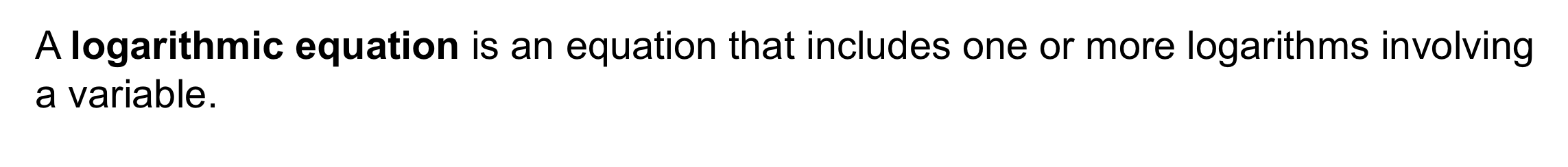11
11
10
Problem 5 Got It?
A
B
C
D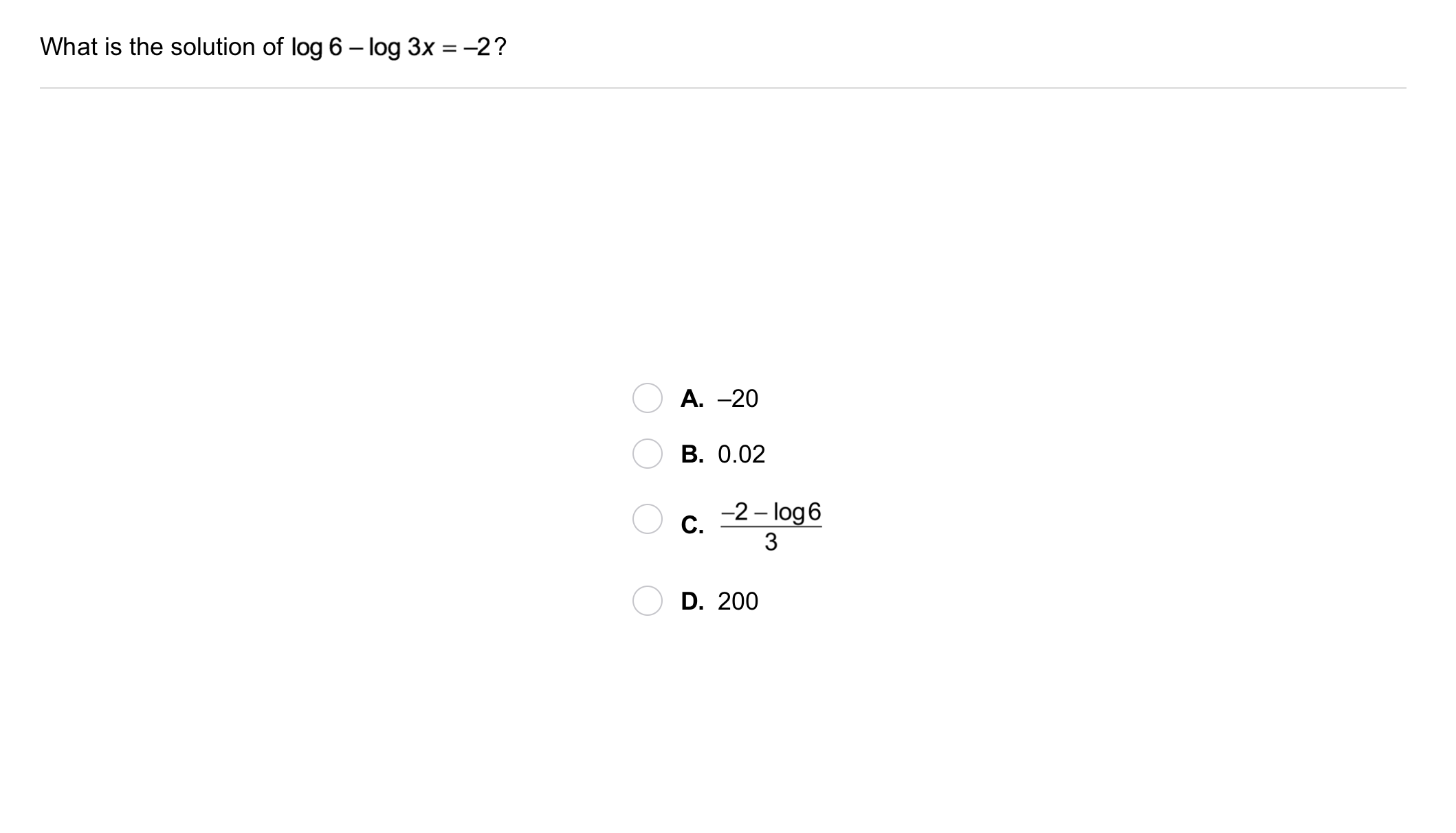12
12
10
Problem 6 Got It?
A
B
C
D13
13
10
Take Note: Summarize the content of this lesson.